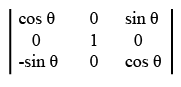# ISRO Scientist or Engineer Electronics 2006

Instructions

For the following questions answer them individually

Question 51

# When a carrier is phase modulated, with an integrated modulating signal, the resultant isQuestion 52

# A satellite orbiting in 600 km orbit transmits 5 GHz frequency. The Doppler shift observed at the ground station, when the satellite is over head of the station isQuestion 53

# A communication channel disturbed by additive white Gaussian noise has a bandwidth of 4kHz and SNR of 15. The highest transmission rate that such a channel can Support (in k-bits/sec) isQuestion 54

# A dual directional coupler is connected in a microwave reflectometer measurement setup. The reading of the power meter in the forward direction is 100 mW and in the reverse direction 4 mw. The VSWR isQuestion 55

# Linear amplifier with a gainof 30dB is fed with $$1.0 \mu W$$ power, the output power of the amplifierQuestion 56

# 10Watts RF Power is transmitted with a circular polarized antenna having gain of 4 0dB. A receiving antenna has vertical polarization The path loss is 100 dB. The receiving signal isQuestion 57

# $$\overrightarrow{P} = 2i - 3j, \overrightarrow{Q} = -3i + 4j - 2k$$, and $$\overrightarrow{R}$$ are in equilibrium, if $$\overrightarrow{R}$$ isQuestion 58

# Arigid bodyis rotating with constant angular velocity $$\omega$$ about a fixed axis, if $$\nu$$ is the velocity of a point of the body, then curl $$\nu =$$Question 59

# Laplace transform of $$\sin^3 2t$$ isQuestion 60

# The value of the determinantOR